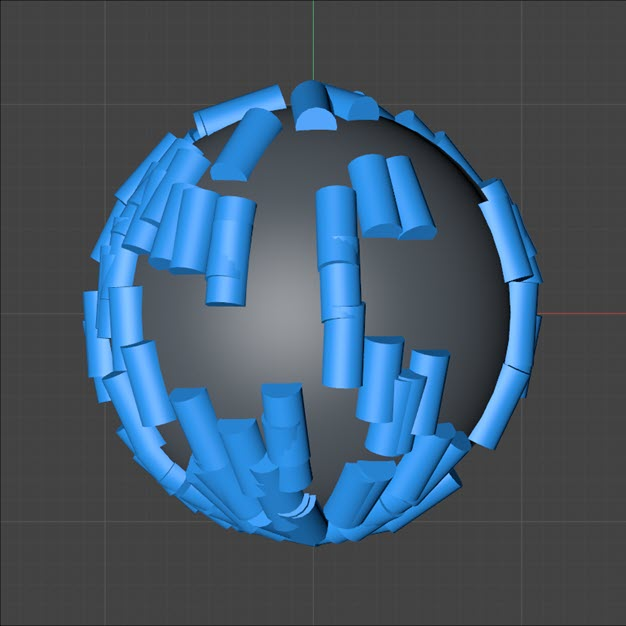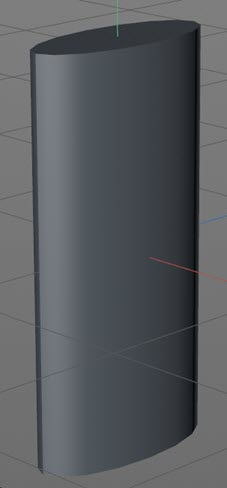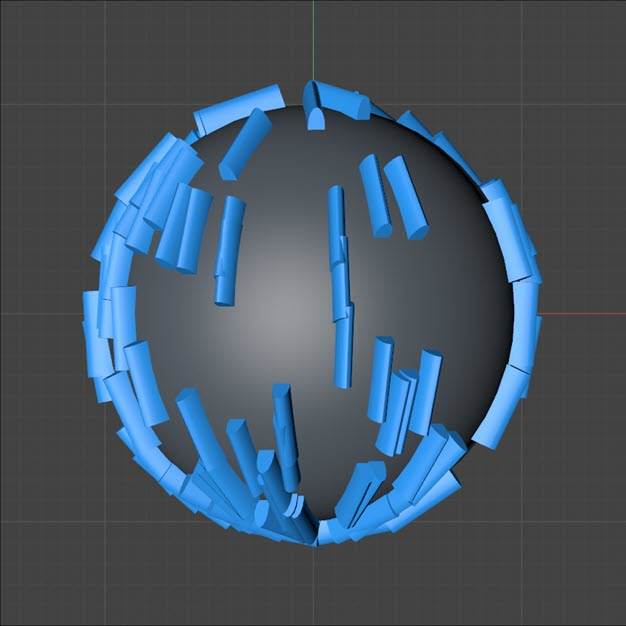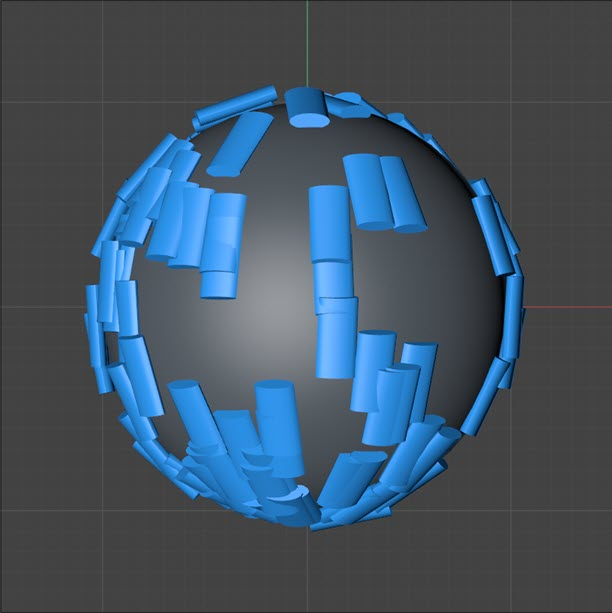# The importance of Tangential Axis

'Tangential Axis' is a setting in the X-Particles emitter 'Extended Data' tab. It is only used when you have enabled rotations and set the rotation to 'Tangential'. Previously, this caused the particle to be rotated so that the Z-axis of the particle was pointing along the particle's direction of travel. If a Generator was linked to the emitter, this would cause the Z-axis of the generated object also to point in the direction of travel.

Note that an identical setting is present in the Spin modifier when set to Rotation mode.

However, this sometimes caused a problem. Suppose the generated object was a primitve Cylinder which by default is oriented so that its height is along the object's Y-axis. In this case the result would look like this if the particles were emitted from a sphere:This might be what was wanted but perhaps we really wanted the cylinder's height to point along the direction of travel. With a Cylinder primitive we can simply change the cylinder's orientation so that the height is aligned along the object's Z-axis, but we can't be sure that we can do this with every object so some objects might be misaligned with no easy way to prevent it.

This is where the 'Tangential Axis' setting comes in. What it does is cause the selected axis to be the one which points along the particle's direction of travel. We can see the effect on a particle in these three images. The object being generated is actually a flattened cylinder with a narrow X-axis, longer Z-axis and the main height along the Y-axis:If 'Tangential Axis' is set to Z:This is the default, which is the same as previous versions. You can see that the longer Z-axis is pointing out from the sphere, showing that the tangential rotation is aligned along the object's Z-axis.

However, the tangential rotation can now be along the X or Y-axes instead. Note that this does not affect the direction of travel, only the particle (and therefore the object) rotation.

If we try the same flattened cylinder again, here it is with 'Tangential Axis' set to the X-axis, Note that the particle has been rotated so that its X-axis points in the direction of travel, so the short X-axis of the object now points in the same direction:And finally with 'Tangential Axis' set to Y, which in this case gives us what we want:You can see that 'Tangential Axis' can be a very useful setting if you need to align any of the object's three axes to point along its direction of travel instead of always the Z-axis. Remember though that this only applies when the particle rotation is set to 'Tangential'.